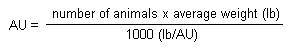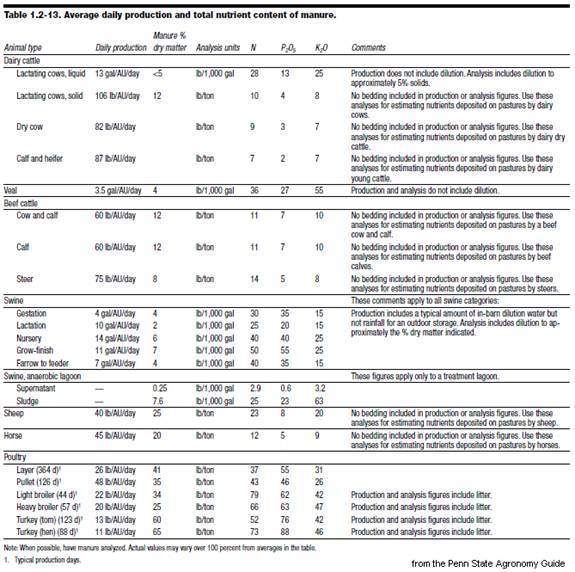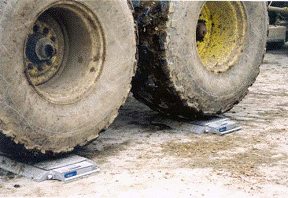## Competency Area 6: Nutrient Management and Planning

### PO 54. Know how to calculate total animal manure production on a livestock farm.

1. Animal excretion plus other additions to process wastewater
Manure quantities and analyses are needed to properly allocate manure to cropland on an annual basis.  Manure production can be measured based on the size of the storage, from records of how many loads of manure of a given size are hauled each year, or from animal inventories and production levels on the farm.

Animal excretion plus other additions to process wastewater uses estimates of per-animal excretion to estimate total manure production.  To use these factors, you need to determine the number of animal units (AU) that are producing manure and the number of days in the manure collection period.  You may need to divide the animals into groups based on different size, age, storage or handling systems, etc.

Animal unit calculation:• For growing animals, use the average weight during the collection period.
• For example: hogs growing from 45 lb to 245 lb would have an average weight of 145 lb.  With 500 pigs: AU = 500 x 145 / 1000 = 72.5 AU
• Use the table (on the next page) to determine daily manure production rates.
• Finishing pigs produce 11 gal manure/AU/day.  Thus for these pigs: 72.5 AU x 11 gal/AU/day = 797.5 gal/day
• This number is multiplied by the number of days that manure is collected.
• If manure is collected and spread every 4 months (120 days): 120 days x 797.5 gal/day = 95,700 gal to spread every 4 months
• Any additions to the manure, such as rainwater, washwater, bedding, etc. should be added to this total for the collection period.Load records and manure spreader calibration calculates manure production based on what has been spread.  There are three methods used to do this.

1. Using truck scales.
• Most accurate, works for solid, semi-solid, and liquid manures.
• Weigh tractor and spreader empty (record each axle weight), and then weigh full.  Full weight – empty weight = manure weight
• Determine application area: measure length and width of application pattern; multiply to get area; and divide by 43,560 (ft2/acre) to get acreage covered.
• Calculate rate: divide manure weight by application acreage; divide this by 2000 to get tons/acre.
2. Method 2: using plastic sheets
• Works for solid and semi-solid manure; requires 3 same-size sheets or tarps and three 5-gallon buckets.
• Determine tarp and pail weight (put one sheet in each bucket; weigh).
• Stake sheets at different intervals along the spread line; apply manure as normal.
• Collect, weigh, and determine rate: put tarp with manure into bucket and weigh; subtracting the original weight of the bucket and tarp.  Take the average of the weights and convert to the rate (see http://nmsp.css.cornell.edu/publications/factsheets/factsheet18.pdf).
 Method 3: counting loads Works for solid, semi-solid, and liquid manures. Requires knowledge of field size and average manure load (tons/load or gal/load).  Apply manure evenly to the whole field, count the loads applied, and calculate rate per acre.Calibrating manure spreader using weights (image source)